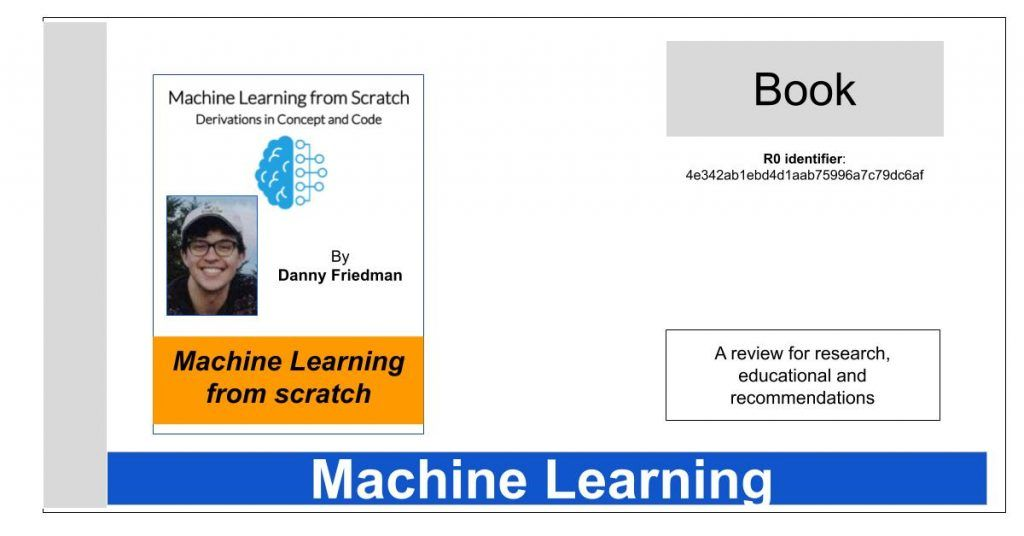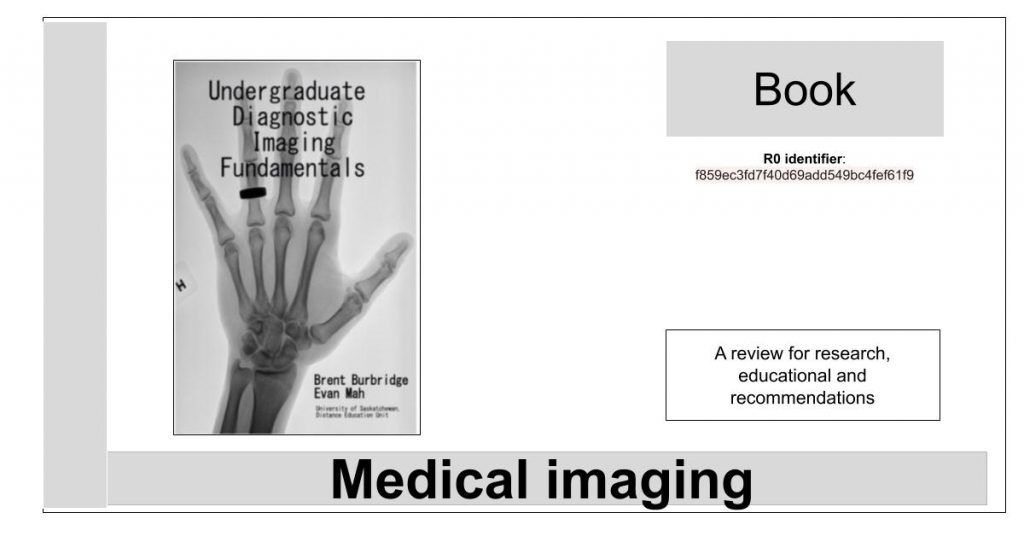# Formación | 🇬🇧 Training## EvalML: a library for automated machine learning and model understanding

EvalML is an AutoML library that builds, optimizes, and evaluates machine learning pipelines using domain-specific objective functions, it is a library for automated machine learning (AutoML) and model understanding, written in Python## Probabilistic Machine Learning: An Introduction

“In this book, we will cover the most common types of ML, but from a probabilistic perspective. Roughly speaking, this means that we treat all unknown quantities (e.g., predictions about the future value of some quantity of interest, such as tomorrow’s temperature, or the parameters of some model) as random variables, that are endowed with probability distributions which describe a weighted set of possible values the variable may have.[…].”.## Data Science: A First Introduction

The book is structured so that learners spend the first four chapters learning how to use the R programming language and Jupyter notebooks to load, wrangle/clean, and visualize data, while answering descriptive and exploratory data analysis questions. The remaining chapters illustrate how to solve four common problems in data science, which are useful for answering predictive and inferential data analysis questions[…]## Bayesian Data Analysis: book & course

This book is intended to have three roles and to serve three associated audiences: an introductory text on Bayesian inference starting from first principles, a graduate text on effective current approaches to Bayesian modeling and computation in statistics and related fields, and a handbook of Bayesian methods in applied statistics for general users of and researchers in applied statistics. Although introductory in its early sections, the book is definitely not elementary in the sense of a first text in statistics## Fourier Neural Operator for Parametric Partial Differential Equations

The classical development of neural networks has primarily focused on learning mappings between finite-dimensional Euclidean spaces. Recently, this has been generalized to neural operators that learn mappings between function spaces. For partial differential equations (PDEs), neural operators directly learn the mapping from any functional parametric dependence to the solution.## Machine Learning from scratch (by Danny Friedman)

This book covers the building blocks of the most common methods in machine learning. This set of methods is like a toolbox for machine learning engineers. Those entering the field of machine learning should feel comfortable with this toolbox so they have the right tool for a variety of tasks.

## OpenAccess Publication System: welcome to OpenScience

We have launched, within the open publishing platform “PubPub“, a research community in English language (its equivalent in Spanish too). The benefit is based on four pillars: 1) readers, 2) authors, 3) reviewers and 4) journals. (This is based in OpenSource software). As part of the Knowledge Futures Group, we’re committed to making PubPub open …